|

# 装修前的强迫症,好过入住后的焦虑症

记得有句话说，无论这个世界上发生什么，都不会影响那些正筹备婚礼的人。这话用在装修房子的人身上大概也合适。

好不容易买（租）到了合适的房子，家装大工程就一刻不停地开始了。明确需求和预算，找设计师，敲定设计稿，请装修队，购买建材、家具家电……每一步都足够让人焦头烂额。假如此时不焦虑，那恐怕等搬进去之后就要焦虑了。看看以下问题，测测你的焦虑程度：

这些你想过吗？

1、 我的房子比较小，能不能从有限的面积里“偷”出较大化的新空间？

2、 我的房子结构怪、采光差/我家有西晒房/我家在南方，回南天特别潮湿，装修的时候还能有办法改善吗？

3、 我对客厅、餐厅、书房这些功能分区还没有特别明确的想法，该怎么确定自己的需求？

4、 我的朋友搬进新家后才发现问题多多，装修前完全没有预见到，我该怎样提前“避坑”呢？

5、 我家有伴侣、有孩子、有老人，还有宠物，怎么能让大家住着“不打架”，满足所有人的需要，甚至能提升家人的关系？

6、 我没有豪宅，可是好羡慕电影、杂志里的“梦幻之家”，这在我的普通房子里能不能实现？

7、 我养狗、会弹钢琴、爱喝红酒、在浴缸泡澡的时候还想玩XBOX，并且我还和婆婆同住……怎么设计装修才能不“委屈”自己呢？

如果你想过这些，那么你至少比大部分只明确了装修预算、其他还一头雾水的房主先行了一步。不过要想真的升级居住体验，只考虑这些问题还远远不够。

好在原点编辑部都替我们想到了。继《这样装修不后悔》《找到家的好感觉》之后，这本《零烦恼居住全书》针对家装过程中我们必然会遇到的100个问题，给出了300种方案，追踪家装全过程，让你变身居住达人。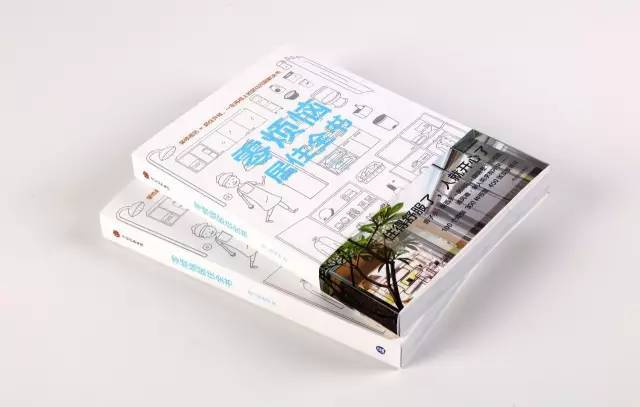家居主题的书一直火热，无数“看上去很美”的家装范例让人艳羡。但是除了让家变得更美更舒适，我们更应该关注一下那些“上不了台面”的问题，例如如何防止蟑螂从水槽里爬出来，抹布放那里合适，鞋柜、马桶、垃圾桶有异味怎么办，和老人共用的洗手间怎样设计最方便……

同样热门的还有收纳的问题，收纳技巧对于提升居家环境非常重要，但是那些急需收纳达人解救的居室，往往是因为装修之初考虑不周，没有设置出充足、合理的储物空间，因此在居住一段时间之后，只好靠见缝插针的收纳窍门来亡羊补牢。

以上这些琐碎的问题，正是需要我们每天面对的，这就是为什么我们需要这本书告诉我们，如何在启动设计装修之前，于每一个细节处未雨绸缪，避免因为前期的思虑不周，给未来生活带来不必要的麻烦。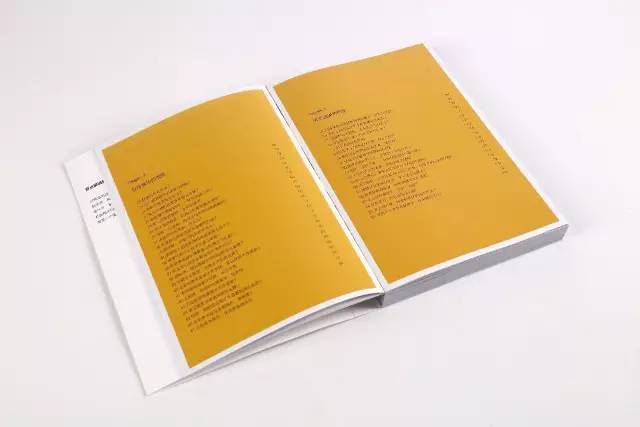有了这些提醒，以后我们就不必面对那些搬进新居之后的尴尬：电源插孔不够用，满地都是电线；厨房预留空间狭窄，两人无法同时操作；漂亮的椅子坐久了让人腰酸背痛；坐轮椅的老人无法通过房门；出门时总找不到钥匙；鞋柜臭臭的；衣柜关门声音太大；浴缸周围的硅胶开始发霉……这些说大不大、说小不小的问题，着实令人烦恼。

在这里，阿信就将这本书中最核心的两个理念介绍给大家，这两个理念已经在某种程度上解决大部分的家装难题了。

一

“动线”决定设计

动线是指人的活动路线。房子住得是否舒适，很大程度上取决于空间动线的设计。好的设计，就是按照居住者的生活习惯和生活方式把空间科学规划起来。空间区隔、家具摆放，甚至小小一个插座、器皿的放置，都要考虑与动线配合。有些动线可以交叉，有些动线一旦交叉，则会使功能区域混乱，浪费住宅空间。

原点编辑部结合大量户型图和实景图，标注出不同使用者、不同功能区内的家居动线，让人一目了然、心中有数。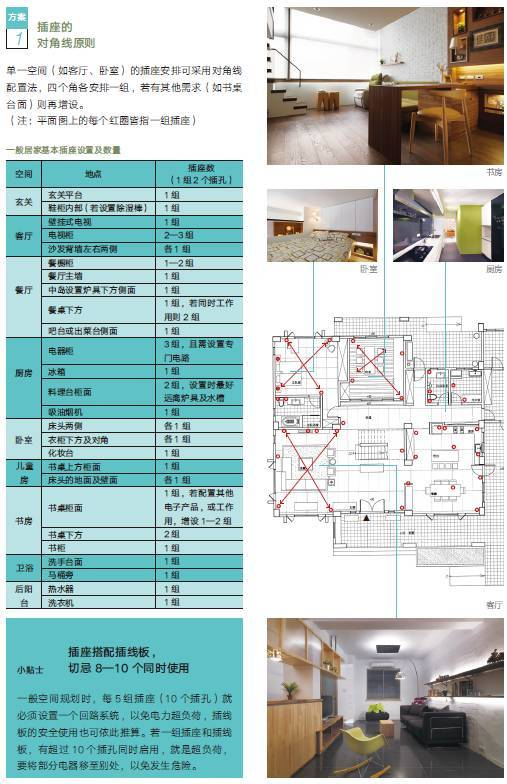除此之外，书中还有充足、科学、精细的数据，标注出合理的空间安排与符合人体工程学的家具尺寸范围，给对数字缺乏概念的家装新手提供参考，非常实用。比如，书桌的深度以60—80厘米为佳，放置电脑显示屏及键盘不会觉得拥挤；若不得已要把书桌的深度控制在50厘米以内，则建议在书桌下方设置键盘抽屉；90厘米以上的宽度较舒适；书桌台面高度则以东方人160—175厘米的身高来计算，80厘米是建议的台面高度，但实际状况必须考虑电脑屏幕大小，一般人坐下来，视线较好能落在屏幕的正中央至屏幕的上缘之间。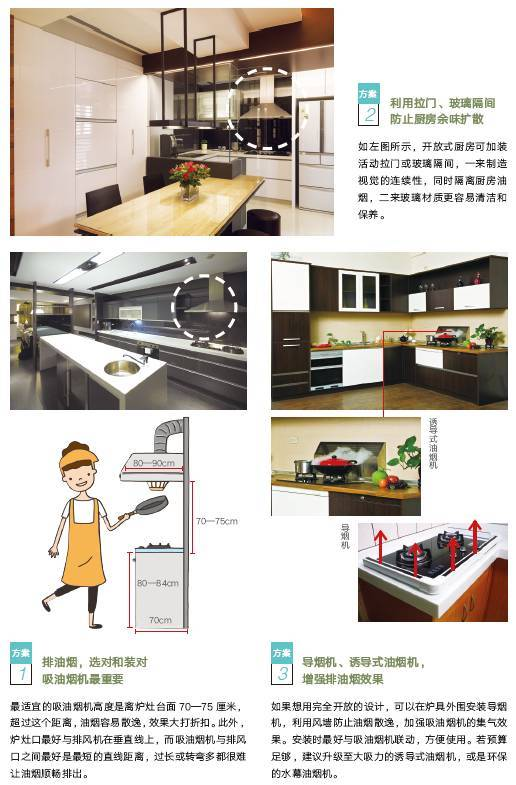二

每个小小的需求都值得被用心对待

在我们的居室中，可能有爱在墙上画画的小朋友，有坐轮椅行动的老人，有和婆婆同住的媳妇，有喜欢用XBOX玩游戏的年轻人，有喜欢邀朋友小酌的人，有信仰宗教的人……

而我们能做的，就是在有限的空间内，尊重每一位居住者的需求：给小朋友设计一堵黑板墙，给老年人设置方便出入的推拉门，给媳妇和婆婆安排互不干扰的厨房空间，给年轻人游戏的空间，给朋友聚会预备一张可伸展餐桌，给需要寄托的心灵开辟一处神明厅……

仅仅一个晒衣的小小后阳台，布置起来都有很多要注意的学问。LED灯的架设让阳台不会阴暗，安装自动或手动式晒衣架，可以轻松又安全地晾衣服，不用因为要经常伸长手臂或脖子而肌肉酸痛。尽量将洗衣机及水槽设计在同一侧，方便处理领口或袖口等细部衣物清洁。

水槽较好要有冷热水，冬天洗衣不怕冷。若有烘衣机，建议架设在洗衣机上方，但要注意使用高度。下方也可收纳洗涤衣物的各式清洁剂。接着再好好利用零星空间、靠墙收挂，让阳台走道维持约60厘米宽的顺畅动线。这样清清爽爽且明亮的后阳台，让人不喜欢得不得了。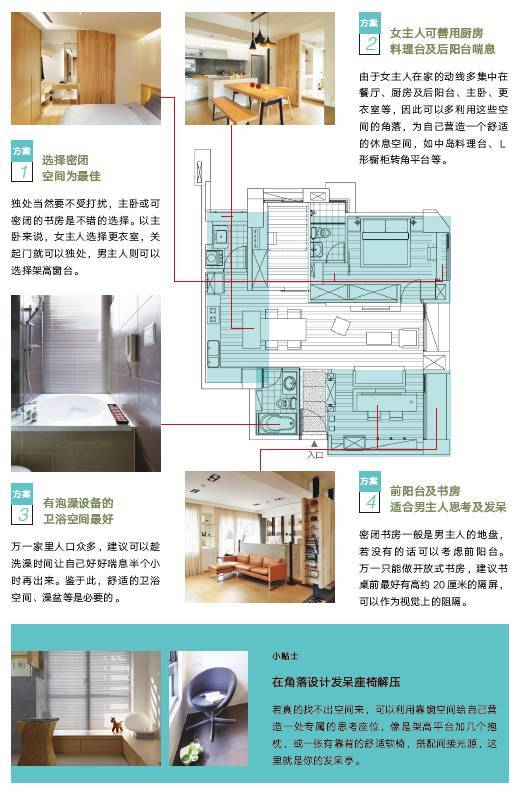在满足所有人的基本需求之外，也别忘了我们那些有点遥远的家居梦想。

开放式厨房是女人们的梦想，但真到了装修时，才知道并非每个家的格局都适合设置开放式厨房，因为有时不只是变动厨房，全家的格局、管线等都要改变，如此一来，装修费会变成原本预估的2—3倍。一般人听到这样的设计及价钱时，装载梦想的热情马上消失了一半，然后继续待在密闭式厨房里与油烟奋斗。有没有少动格局就能拥有开放式厨房的方法呢？答案是有的。方法还不少。包括在厨房及餐厅开窗，设计一个出菜台；将餐厅及厨房的隔间墙拿掉一半，成为隔屏；将厨房与餐厅结合、把厨房改成储藏室，等等。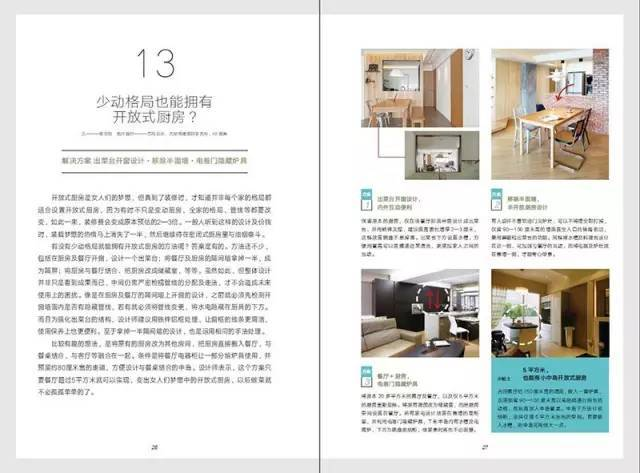家装时较大的误区，是概念先行，忽略了装修布置的真正目的是为了每天生活其中。所有的家装细节，都要较大程度适应我们原有的生活习惯和需求，而不是给未来制造麻烦。

居住的幸福指数，从来不是靠简单的收纳可以改善的。单纯的断舍离，或者把一切装进收纳盒并不管用。想要实现所有期待，就该先抛弃那些条条框框，在家装中融入巧妙的心思。

我们的居室承担着太多的功能。我们每天在这里饮食、休憩、清洁，也会在这里工作、运动、娱乐、社交。家收纳我们的物品，保留我们的私隐，也为我们提供寄托心灵的空间。

居室就是人，人即居室。

`声明：本文由入驻焦点开放平台的作者撰写，除焦点官方账号外，观点仅代表作者本人，不代表焦点立场错误信息举报电话： 400-099-0099，邮箱：jubao@vip.sohu.com，或点此进行意见反馈，或点此进行举报投诉。`A B C D E F G H J K L M N P Q R S T W X Y Z
A - B - C - D - E
• A
• 鞍山
• 安庆
• 安阳
• 安顺
• 安康
• 澳门
• B
• 北京
• 保定
• 包头
• 巴彦淖尔
• 本溪
• 蚌埠
• 亳州
• 滨州
• 北海
• 百色
• 巴中
• 毕节
• 保山
• 宝鸡
• 白银
• 巴州
• C
• 承德
• 沧州
• 长治
• 赤峰
• 朝阳
• 长春
• 常州
• 滁州
• 池州
• 长沙
• 常德
• 郴州
• 潮州
• 崇左
• 重庆
• 成都
• 楚雄
• 昌都
• 慈溪
• 常熟
• D
• 大同
• 大连
• 丹东
• 大庆
• 东营
• 德州
• 东莞
• 德阳
• 达州
• 大理
• 德宏
• 定西
• 儋州
• 东平
• E
• 鄂尔多斯
• 鄂州
• 恩施
F - G - H - I - J
• F
• 抚顺
• 阜新
• 阜阳
• 福州
• 抚州
• 佛山
• 防城港
• G
• 赣州
• 广州
• 桂林
• 贵港
• 广元
• 广安
• 贵阳
• 固原
• H
• 邯郸
• 衡水
• 呼和浩特
• 呼伦贝尔
• 葫芦岛
• 哈尔滨
• 黑河
• 淮安
• 杭州
• 湖州
• 合肥
• 淮南
• 淮北
• 黄山
• 菏泽
• 鹤壁
• 黄石
• 黄冈
• 衡阳
• 怀化
• 惠州
• 河源
• 贺州
• 河池
• 海口
• 红河
• 汉中
• 海东
• 怀来
• I
• J
• 晋中
• 锦州
• 吉林
• 鸡西
• 佳木斯
• 嘉兴
• 金华
• 景德镇
• 九江
• 吉安
• 济南
• 济宁
• 焦作
• 荆门
• 荆州
• 江门
• 揭阳
• 金昌
• 酒泉
• 嘉峪关
K - L - M - N - P
• K
• 开封
• 昆明
• 昆山
• L
• 廊坊
• 临汾
• 辽阳
• 连云港
• 丽水
• 六安
• 龙岩
• 莱芜
• 临沂
• 聊城
• 洛阳
• 漯河
• 娄底
• 柳州
• 来宾
• 泸州
• 乐山
• 六盘水
• 丽江
• 临沧
• 拉萨
• 林芝
• 兰州
• 陇南
• M
• 牡丹江
• 马鞍山
• 茂名
• 梅州
• 绵阳
• 眉山
• N
• 南京
• 南通
• 宁波
• 南平
• 宁德
• 南昌
• 南阳
• 南宁
• 内江
• 南充
• P
• 盘锦
• 莆田
• 平顶山
• 濮阳
• 攀枝花
• 普洱
• 平凉
Q - R - S - T - W
• Q
• 秦皇岛
• 齐齐哈尔
• 衢州
• 泉州
• 青岛
• 清远
• 钦州
• 黔南
• 曲靖
• 庆阳
• R
• 日照
• 日喀则
• S
• 石家庄
• 沈阳
• 双鸭山
• 绥化
• 上海
• 苏州
• 宿迁
• 绍兴
• 宿州
• 三明
• 上饶
• 三门峡
• 商丘
• 十堰
• 随州
• 邵阳
• 韶关
• 深圳
• 汕头
• 汕尾
• 三亚
• 三沙
• 遂宁
• 山南
• 商洛
• 石嘴山
• T
• 天津
• 唐山
• 太原
• 通辽
• 铁岭
• 泰州
• 台州
• 铜陵
• 泰安
• 铜仁
• 铜川
• 天水
• 天门
• W
• 乌海
• 乌兰察布
• 无锡
• 温州
• 芜湖
• 潍坊
• 威海
• 武汉
• 梧州
• 渭南
• 武威
• 吴忠
• 乌鲁木齐
X - Y - Z
• X
• 邢台
• 徐州
• 宣城
• 厦门
• 新乡
• 许昌
• 信阳
• 襄阳
• 孝感
• 咸宁
• 湘潭
• 湘西
• 西双版纳
• 西安
• 咸阳
• 西宁
• 仙桃
• 西昌
• Y
• 阳泉
• 运城
• 营口
• 盐城
• 扬州
• 鹰潭
• 宜春
• 烟台
• 宜昌
• 岳阳
• 益阳
• 永州
• 阳江
• 云浮
• 玉林
• 宜宾
• 雅安
• 玉溪
• 延安
• 榆林
• 银川
• Z
• 张家口
• 镇江
• 舟山
• 漳州
• 淄博
• 枣庄
• 郑州
• 周口
• 驻马店
• 株洲
• 张家界
• 珠海
• 湛江
• 肇庆
• 中山
• 自贡
• 资阳
• 遵义
• 昭通
• 张掖
• 中卫

1室1厅1厨1卫1阳台

1
2
3
4
5

0
1
2

1

1

0
1
2
3报名成功，资料已提交审核A B C D E F G H J K L M N P Q R S T W X Y Z
A - B - C - D - E
• A
• 鞍山
• 安庆
• 安阳
• 安顺
• 安康
• 澳门
• B
• 北京
• 保定
• 包头
• 巴彦淖尔
• 本溪
• 蚌埠
• 亳州
• 滨州
• 北海
• 百色
• 巴中
• 毕节
• 保山
• 宝鸡
• 白银
• 巴州
• C
• 承德
• 沧州
• 长治
• 赤峰
• 朝阳
• 长春
• 常州
• 滁州
• 池州
• 长沙
• 常德
• 郴州
• 潮州
• 崇左
• 重庆
• 成都
• 楚雄
• 昌都
• 慈溪
• 常熟
• D
• 大同
• 大连
• 丹东
• 大庆
• 东营
• 德州
• 东莞
• 德阳
• 达州
• 大理
• 德宏
• 定西
• 儋州
• 东平
• E
• 鄂尔多斯
• 鄂州
• 恩施
F - G - H - I - J
• F
• 抚顺
• 阜新
• 阜阳
• 福州
• 抚州
• 佛山
• 防城港
• G
• 赣州
• 广州
• 桂林
• 贵港
• 广元
• 广安
• 贵阳
• 固原
• H
• 邯郸
• 衡水
• 呼和浩特
• 呼伦贝尔
• 葫芦岛
• 哈尔滨
• 黑河
• 淮安
• 杭州
• 湖州
• 合肥
• 淮南
• 淮北
• 黄山
• 菏泽
• 鹤壁
• 黄石
• 黄冈
• 衡阳
• 怀化
• 惠州
• 河源
• 贺州
• 河池
• 海口
• 红河
• 汉中
• 海东
• 怀来
• I
• J
• 晋中
• 锦州
• 吉林
• 鸡西
• 佳木斯
• 嘉兴
• 金华
• 景德镇
• 九江
• 吉安
• 济南
• 济宁
• 焦作
• 荆门
• 荆州
• 江门
• 揭阳
• 金昌
• 酒泉
• 嘉峪关
K - L - M - N - P
• K
• 开封
• 昆明
• 昆山
• L
• 廊坊
• 临汾
• 辽阳
• 连云港
• 丽水
• 六安
• 龙岩
• 莱芜
• 临沂
• 聊城
• 洛阳
• 漯河
• 娄底
• 柳州
• 来宾
• 泸州
• 乐山
• 六盘水
• 丽江
• 临沧
• 拉萨
• 林芝
• 兰州
• 陇南
• M
• 牡丹江
• 马鞍山
• 茂名
• 梅州
• 绵阳
• 眉山
• N
• 南京
• 南通
• 宁波
• 南平
• 宁德
• 南昌
• 南阳
• 南宁
• 内江
• 南充
• P
• 盘锦
• 莆田
• 平顶山
• 濮阳
• 攀枝花
• 普洱
• 平凉
Q - R - S - T - W
• Q
• 秦皇岛
• 齐齐哈尔
• 衢州
• 泉州
• 青岛
• 清远
• 钦州
• 黔南
• 曲靖
• 庆阳
• R
• 日照
• 日喀则
• S
• 石家庄
• 沈阳
• 双鸭山
• 绥化
• 上海
• 苏州
• 宿迁
• 绍兴
• 宿州
• 三明
• 上饶
• 三门峡
• 商丘
• 十堰
• 随州
• 邵阳
• 韶关
• 深圳
• 汕头
• 汕尾
• 三亚
• 三沙
• 遂宁
• 山南
• 商洛
• 石嘴山
• T
• 天津
• 唐山
• 太原
• 通辽
• 铁岭
• 泰州
• 台州
• 铜陵
• 泰安
• 铜仁
• 铜川
• 天水
• 天门
• W
• 乌海
• 乌兰察布
• 无锡
• 温州
• 芜湖
• 潍坊
• 威海
• 武汉
• 梧州
• 渭南
• 武威
• 吴忠
• 乌鲁木齐
X - Y - Z
• X
• 邢台
• 徐州
• 宣城
• 厦门
• 新乡
• 许昌
• 信阳
• 襄阳
• 孝感
• 咸宁
• 湘潭
• 湘西
• 西双版纳
• 西安
• 咸阳
• 西宁
• 仙桃
• 西昌
• Y
• 阳泉
• 运城
• 营口
• 盐城
• 扬州
• 鹰潭
• 宜春
• 烟台
• 宜昌
• 岳阳
• 益阳
• 永州
• 阳江
• 云浮
• 玉林
• 宜宾
• 雅安
• 玉溪
• 延安
• 榆林
• 银川
• Z
• 张家口
• 镇江
• 舟山
• 漳州
• 淄博
• 枣庄
• 郑州
• 周口
• 驻马店
• 株洲
• 张家界
• 珠海
• 湛江
• 肇庆
• 中山
• 自贡
• 资阳
• 遵义
• 昭通
• 张掖
• 中卫• 手机• 分享
• 设计
免费设计
• 计算器
装修计算器
• 联系
• 置顶
返回顶部